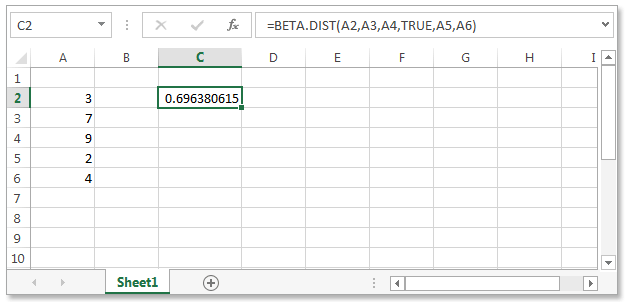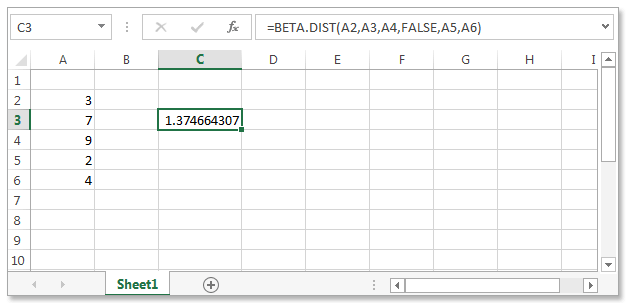# Excel BETA.DIST() function

## BETA.DIST()

The beta distribution (BETA.DIST() function is used to study variation in the percentage of something across samples, such as the fraction of the day people spend watching football matches on television.

Version: Excel 2013

Syntax

```BETA.DIST(x,alpha,beta,cumulative,[A],[B])
```

Parameters:

Name Description Data Category Required/
Optional
X The value between A and B at which to evaluate the function number Required
Alpha A parameter of the distribution. number Required
Beta A parameter of the distribution. number Required
Cumulative A logical value that determines the form of the function.
If cumulative is TRUE, BETA.DIST returns the cumulative distribution function;
if FALSE, it returns the probability density function.
boolean Required
A A lower bound to the interval of x. number Optional
B An upper bound to the interval of x. number Optional

Return Values:

• If any argument is nonnumeric, BETA.DIST returns the #VALUE! error value.
• If alpha ≤ 0 or beta ≤ 0, BETA.DIST returns the #NUM! error value.
• If x < A, x > B, or A = B, BETA.DIST returns the #NUM! error value.
• If you omit values for A and B, BETA.DIST uses the standard cumulative beta distribution so that A = 0 and B = 1

Example - 1 : Excel BETA.DIST() function
In the following worksheet BETA.DIST returns the cumulative distribution function (cumulative is TRUE) for the data present in sample data column in the table.

Sample Data Description
3 Value at which to evaluate the function
7 Parameter of the distribution
9 Parameter of the distribution
2 Lower bound
4 Upper boundExample - 2:

In the following example BETA.DIST returns the probability density function (cumulative is FALSE) for the above sample data :Previous: Mathematical Functions - Excel 2013
Next: Excel DATE() function

﻿